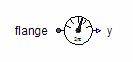Harmonic - MapleSim Help

Harmonic

Compute the harmonicDescription The Harmonic component computes the element-wise magnitude and phase of a single harmonic over the period of a specified frequency.

Connections

 Name Description Modelica ID $u$ Real input signal u ${y}_{\mathrm{rms}}$ Root mean square of polar representation y_rms ${y}_{\mathrm{arg}}$ Angle of polar representation y_arg

Parameters

 Name Default Units Description Modelica ID $f$ $50$ $\mathrm{Hz}$ Base frequency f $k$ $1$ Order of harmonic k use complex conjugate $\mathrm{false}$ Gives conjugate complex result if true useComplexConjugate $\mathrm{x0Cos}$ $0$ Start value of cos integrator state x0Cos $\mathrm{x0Sin}$ $0$ Start value of sin integrator state x0Sin

 Modelica Standard Library The component described in this topic is from the Modelica Standard Library. To view the original documentation, which includes author and copyright information, click here.